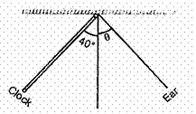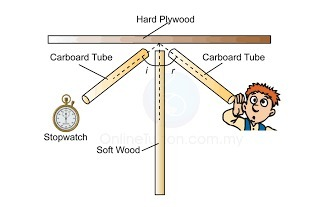### Sound - Test Papers

CBSE Test Paper 01

Chapter 12 Sound

1. The frequency of a visible light of wavelength 600 nm is (speed of light = 3 ×108 m/s), (1nm = 10-9 m) (1)
1. 50 Hz
2. $×$ 1014 Hz
3. 0.5 $×$ 1016 Hz
4. 500 Hz
2. A pulse (1)
1. is a short duration disturbance
2. does not repeat
3. all of these
4. can travel
3. In which of the following medium, speed of sound is maximum? (1)
1. Aluminium
2. Water
3. Air
4. Helium
4. Infrasonic sound is produced by:- (1)
1. Rhinoceros
2. Dogs
3. Rats
4. Bats
5. Which wave property determines loudness? (1)
1. amplitude
2. pitch
3. frequency
4. All of these
6. Sometimes sound produced by bats, dolphins are (1)
1. all of these
2. ultrasonic
3. ultraviolet
4. infrasonic
7. The audible range of frequencies for a normal human being is _____ to _______ Hertz. (1)
1. 30 to 30,000
2. 20 to 20,000
3. 40 to 40,000
4. 10 to 10,000
8. What is the audible range of the average human ear? (1)

9. What is one complete oscillation? (1)

10. In the experiment on studying the laws of reflection of sound, the tube facing the clock is placed as shown. The position of the second tube, at which the ear will get the best reflected sound, is obtained when θequals: (1)11. Calculate the wavelength of a sound wave whose frequency is 220 Hz and speed is 440 m/s in a given medium. (3)

12. How do bats use ultrasound to catch a prey? (3)
13. What is reverberation? What is done to reduce it? (3)

14. A sound wave of wavelength 0.332 m has a time period of 10-3s. If the time period is decreased to 10-4s, calculate the wavelength and frequency of new wave. (3)

1. What is meant by reflection of sound?
2. Describe an activity to study the reflection of sound.
3. State the laws of reflection of sound. (5)

CBSE Test Paper 01
Chapter 12 Sound

1. 5 × 1014 Hz
Explanation: The wavelength (λ) times frequency (ν) equals the speed of light (c), which is 3.00×108m/s to three significant figures.
c = λ⋅ν
ν = c/λ
Frequency is measured in Hertz (Hz), which is /s or s−1.
Convert 600 nm to meters since the unit for the speed of light is m/s.
1 nm=1×10−9m
600nm×1×10−9m1nm=6×10−7m$600nm×\frac{1×{10}^{-9}\phantom{\rule{thickmathspace}{0ex}}m}{1}\phantom{\rule{thickmathspace}{0ex}}nm=6×{10}^{-7}m$V=cλ$V=\frac{c}{\lambda }$V=3×108m/s6×10−7m$\phantom{\rule{0ex}{0ex}}V\phantom{\rule{thickmathspace}{0ex}}=\phantom{\rule{thickmathspace}{0ex}}\frac{3×\phantom{\rule{thickmathspace}{0ex}}{10}^{8}\phantom{\rule{thickmathspace}{0ex}}m/s}{6\phantom{\rule{thickmathspace}{0ex}}×{10}^{-7}m}\phantom{\rule{thickmathspace}{0ex}}$=0.5×1015=5×1014Hz$=\phantom{\rule{thickmathspace}{0ex}}0.5\phantom{\rule{thickmathspace}{0ex}}×\phantom{\rule{thickmathspace}{0ex}}{10}^{15}\phantom{\rule{thickmathspace}{0ex}}=5\phantom{\rule{thickmathspace}{0ex}}\phantom{\rule{thickmathspace}{0ex}}×\phantom{\rule{thickmathspace}{0ex}}{10}^{14}Hz$
1. all of these
Explanation: Pulse is a short duration disturbance. .Pulse does not repeat. Pulse is formed in small portion and Pulse disturbance is momentary.
1. Aluminium
Explanation: The speed of sound is faster in solid medium in comparison to liquid and gaseous medium. Aluminium is solid and metal, so the speed of sound will be maximum in it. Speed of sound in Aluminium at 25 degree celcius is 6420 ms-1
1. Rhinoceros
Explanation: Rhinoceroses communicate with one another by using infrasonic sound of frequency as low as 5 Hz.
1. amplitude
Explanation: Loudness of sound depends upon amplitude,more the amplitude of a wave, more is the loudness produced. Amplitude is the maximum displacement of a particle from its mean position.
1. ultrasonic
Explanation: Sound of frequencies above 20,000 Hz is known as ultrasonic which are produced by bats and dolphins.
1. 20 to 20,000
Explanation: The audible range of an average human ear lies between 20 Hz to 20,000 Hz. Humans cannot hear sounds having frequency less than 20 Hz and greater than 20,000 Hz.
1. 20 Hz to 20,000Hz.
2. The change in density from one maximum value to the minimum value and again to the maximum value makes one complete oscillation.
3. According to law of reflection $\mathrm{\angle }$i = $\mathrm{\angle }$r
Since $\mathrm{\angle }$i = 40o$\theta$ the angle of reflection should be 40o
4. Since we know $v=n×\lambda$
$440=220×\lambda$
$\lambda$ = 440/220 = 2 m
5. Bats emit high pitched ultrasonic squeaks while flying and listen to the echoes produced by the reflection of their squeaks from the prey and returned to bat’s ear as shown in figure. The nature of reflections tells the bat where the obstacle or prey is and what it is like.
6. The repeated reflection of sound that results in the persistence of sound is called as reverberation. We can reduce reverberation by:-
1) Covering roofs and walls of auditorium with sound absorbing materials.
2) seat materials is also selected on the basis of sound absorbing property.
7. Given wavelength, $\lambda$= 0.332 m
Time period of wave, 10-3s
Therefore, Frequency of wave, v = $\frac{1}{T}$
$\frac{1}{{10}^{-3}}$
= 1000 Hz
Therefore, Velocity of wave, $\upsilon =v\lambda$= 1000 $×$ 0.332 = 332 m/s
In a given medium, the velocity of sound wave remains same.
New time period of new wave, T’= 10-4s
Frequency of new wave, v' = $\frac{1}{{T}^{\prime }}$
$\frac{1}{{10}^{-4}}$
= 10,000 Hz
Therefore, Wavelength of new wave, ${\lambda }^{\prime }=\frac{\upsilon }{{v}^{\prime }}$ = $\frac{332}{10,000}$
= 0.0332 m
8. i) The phenomenon of bouncing back of a sound wave from a surface (i.e. going from one medium to the same medium after striking the second medium) is known as reflection of sound.
ii) Activity:
1. Take two identical pipes, as shown in figure.
2. The length of the pipes should be sufficiently long.
3. Arrange them on a table near a wall.
4. Put a clock near the open end of the one pipe as shown in figure.
5. Now, try to hear the sound through the other pipe.
6. Adjust the position of the pipe to hear best sound of clock.
7. Now, measure the angles of incidence and reflection and see the relationship between the angles.
8. The angle of incidence and the angle of reflection of sound are found to be equal.
9. Also, Tubes are placed normal to the vertical reflector, and the reflected wave lie on the same plane. Hence law of reflection are verified.iii) Laws of reflection:
1. The angle of incidence of sound wave is always equal to the angle of reflection of sound wave.
2. The incident sound wave, reflected sound wave and the normal to the reflecting surface at the point of incidence, all lie in the same plane.